1.不要浪费大量的时间在获得样本上。实际上，太多的样本数并不会使学习算法更加的优秀。要尝试调整你的系数：

1.使用更少的特征。

2.增加多项式。

3.调整$\lambda$。

2.诊断学习算法：

1.将样本打乱，并将其中一部分作为训练样本，剩下的作为测试样本，来判断是否出现了过拟合。

2.将样本划分为三类：训练集，交叉验证(cross validation,cv)集，测试集。对于线性回归，训练集来得出相应次数下的theta，交叉验证集来比较这些theta，获得最好的模型，测试集继续训练模型。

1.支持向量机(support vector machine,SVM)：一种监督分类模型。它的目的是寻找一个超平面来对样本进行分割，分割的原则是间隔最大化，最终转化为一个凸二次规划问题来求解。

cost函数：$cost_1(x)=max(0,-\theta^{T}x+1)$

$cost_0(x)=max(0,\theta^{T}x+1)$

J函数：$J_{\theta}(x)=\sum_{i=1}^{m}(y^{(i)}cost_1(\theta^{T}x^{(i)})+(1-y^{(i)})cost_0(\theta^{T}x^{(i)}))+\lambda\sum_{i=1}^{n}{\theta_i^2}$

h函数：$h_{\theta}(x)=\begin{cases}1\quad if\quad \theta^{T}x\geq0\\0\quad otherwise\end{cases}$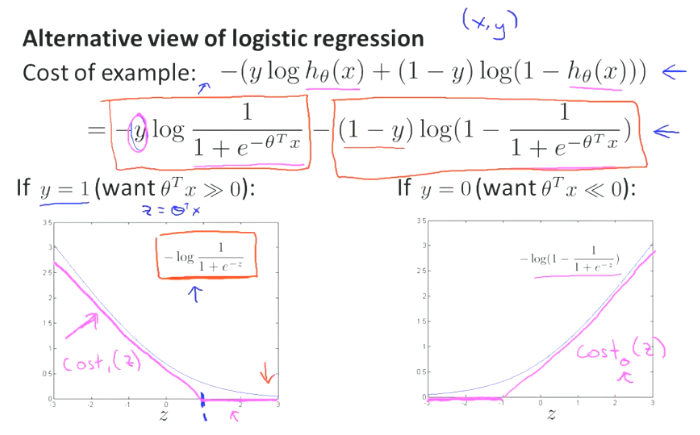SVM在做什么？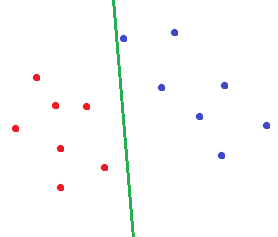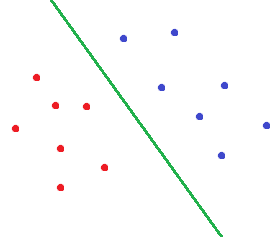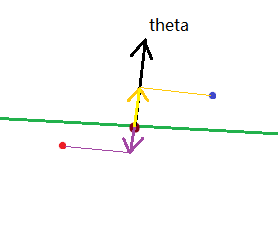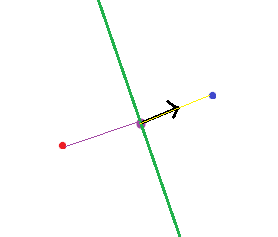2.高斯核函数(Gaussian kernal)：

$f_i$：第i个特征组合，为了方便表示像x1x2,x1x2x3这样的式子。

$K(x,l^{(i)})=exp(-\frac{||x-l^{(i)}||^2}{2\sigma^2})$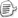posted on 2020-02-21 18:44  GreenDuck  阅读(...)  评论(...编辑  收藏